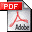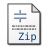Sparse Signal Reconstruction from Quantized Noisy Measurements via GEM Hard Thresholding

Authors:
Kun Qiu and Aleksandar Dogandžić

Reference:
IEEE Trans. Signal Processing, vol. 60, pp. 2628-2634, May 2012.

Abstract:
We develop a generalized expectation-maximization (GEM) algorithm for sparse signal reconstruction from quantized noisy measurements. The measurements follow an underdetermined linear model with sparse regression coefficients, corrupted by additive white Gaussian noise having unknown variance. These measurements are quantized into bins and only the bin indices are used for reconstruction. We treat the unquantized measurements as the missing data and propose a GEM iteration that aims at maximizing the likelihood function with respect to the unknown parameters. Under mild conditions, our GEM iteration yields a convergent monotonically non-decreasing likelihood function sequence and the Euclidean distance between two consecutive GEM signal iterates goes to zero as the number of iterations grows. We compare the proposed scheme with the state-of-the-art convex relaxation method for quantized compressed sensing via numerical simulations.

Index Terms âCompressed sensing, generalized expectation-maximization (GEM) algorithm, quantization, sparse signal reconstruction.